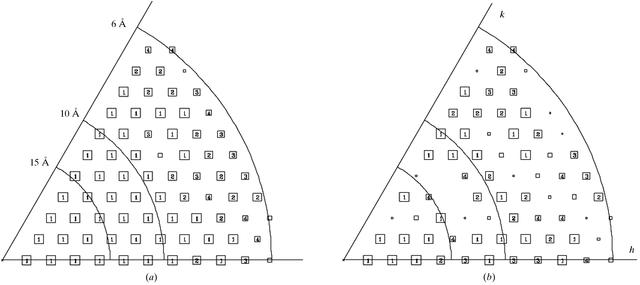disable zoom     view article Figure 2 Combined phase errors as a measure of projection data quality. As shown in Fig. 1, neither the phase error obtained from averaging of the data nor the deviation of the average phase angles from applicable theoretical target values provide a straightforward measure of data quality in plane groups with twofold symmetry along the z axis. In these cases, the presentation of `combined phase errors' may be the best solution to provide a complete account of data quality. Data from the gap-junction specimen were used to compile Fig. 2. Shown in (a) is the FOM for each reflection obtained by data averaging using the program AVRGAMPHS. The corresponding `combined phase error' for each reflection is presented in (b). The `combined phase error' of a reflection is defined as `combined error' (°) = cos−1(FOM*cosαav), where FOM is the figure of merit (ranging from 0 to 1) and αav is the average phase angle after data averaging. Both FOM and αav are calculated by AVRGAMPHS. According to its definition, the `combined phase error' reflects both the statistical significance of the averaged phase angle of a reflection and its deviation from the applicable target phase value. Furthermore, the term (FOM*cosαav) normally serves as a weight for the structure factors if centrosymmetric phase constraints are enforced in the Fourier summation. This procedure minimizes the contribution of unreliable reflections. For plotting purposes, increasing errors are represented by decreasing box sizes. Numbers inside the boxes are assigned to phase errors as follows, 1 < 8°, 2 < 14°, 3 < 20°, 4 < 30°. No numbers are written out for errors encoded by the small box sizes, 5 < 40°, 6 < 50°, 7 < 70° and 8 < 90°. In both representations, an error of 90° indicates that the phase of the reflection is not significant.BIOLOGICALCRYSTALLOGRAPHY
ISSN: 1399-0047
Volume 56| Part 10| October 2000| Pages 1259-1269